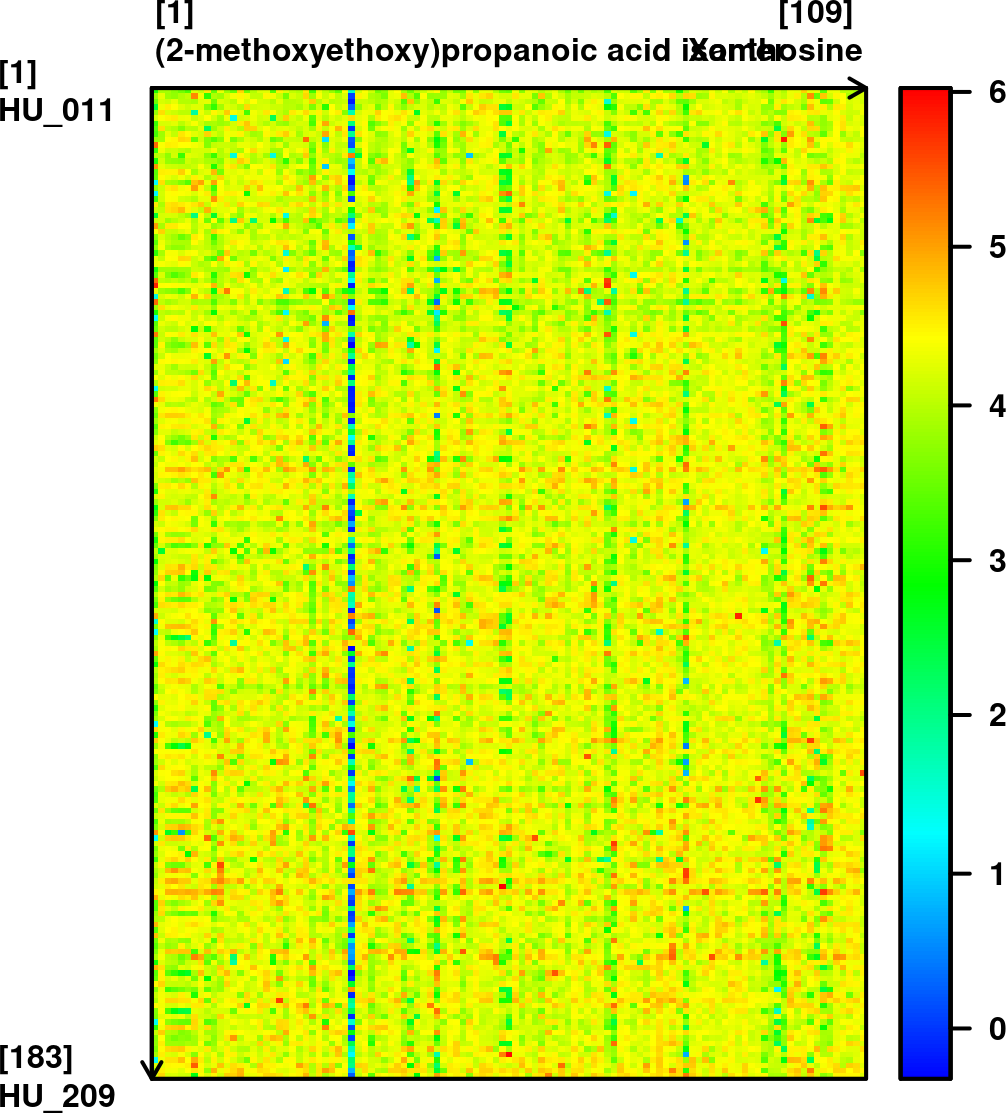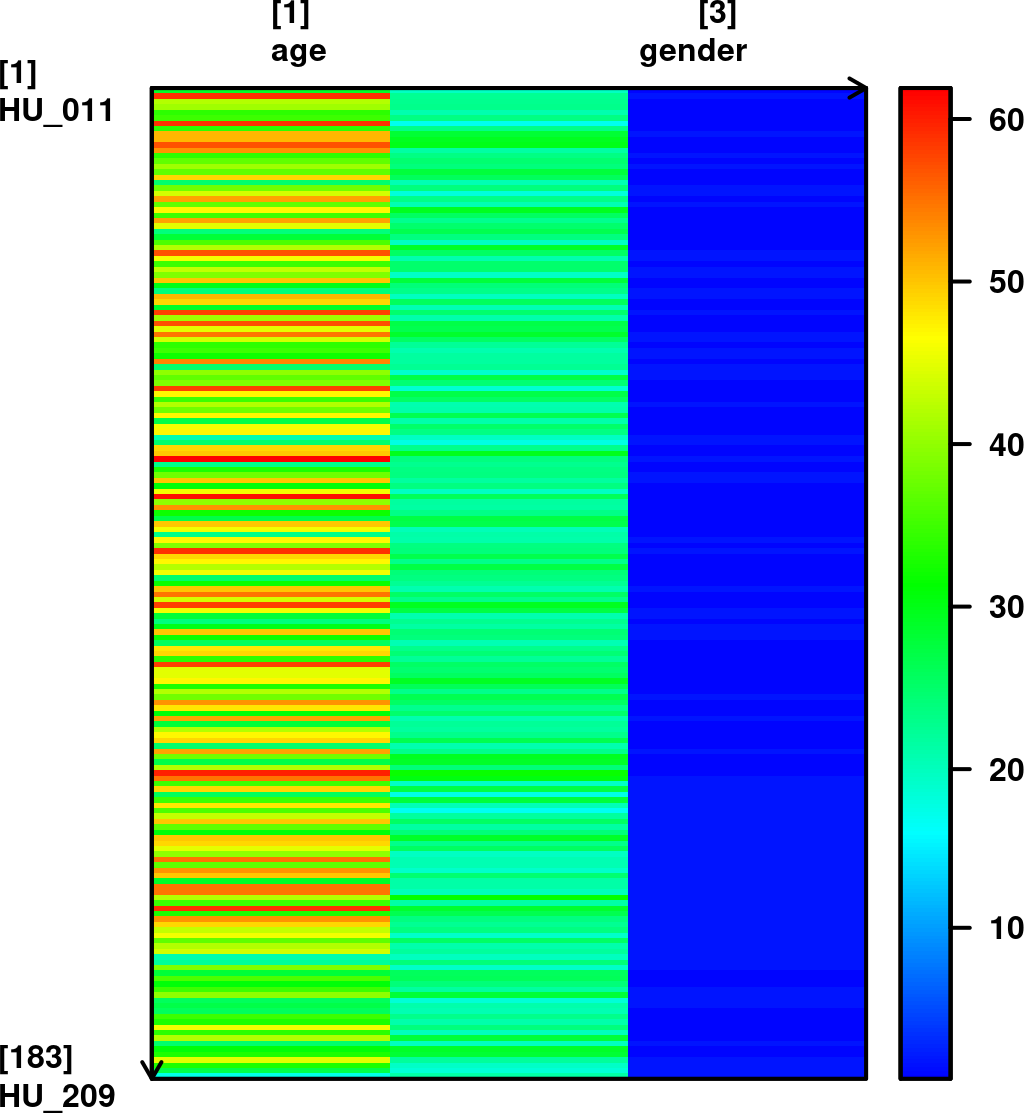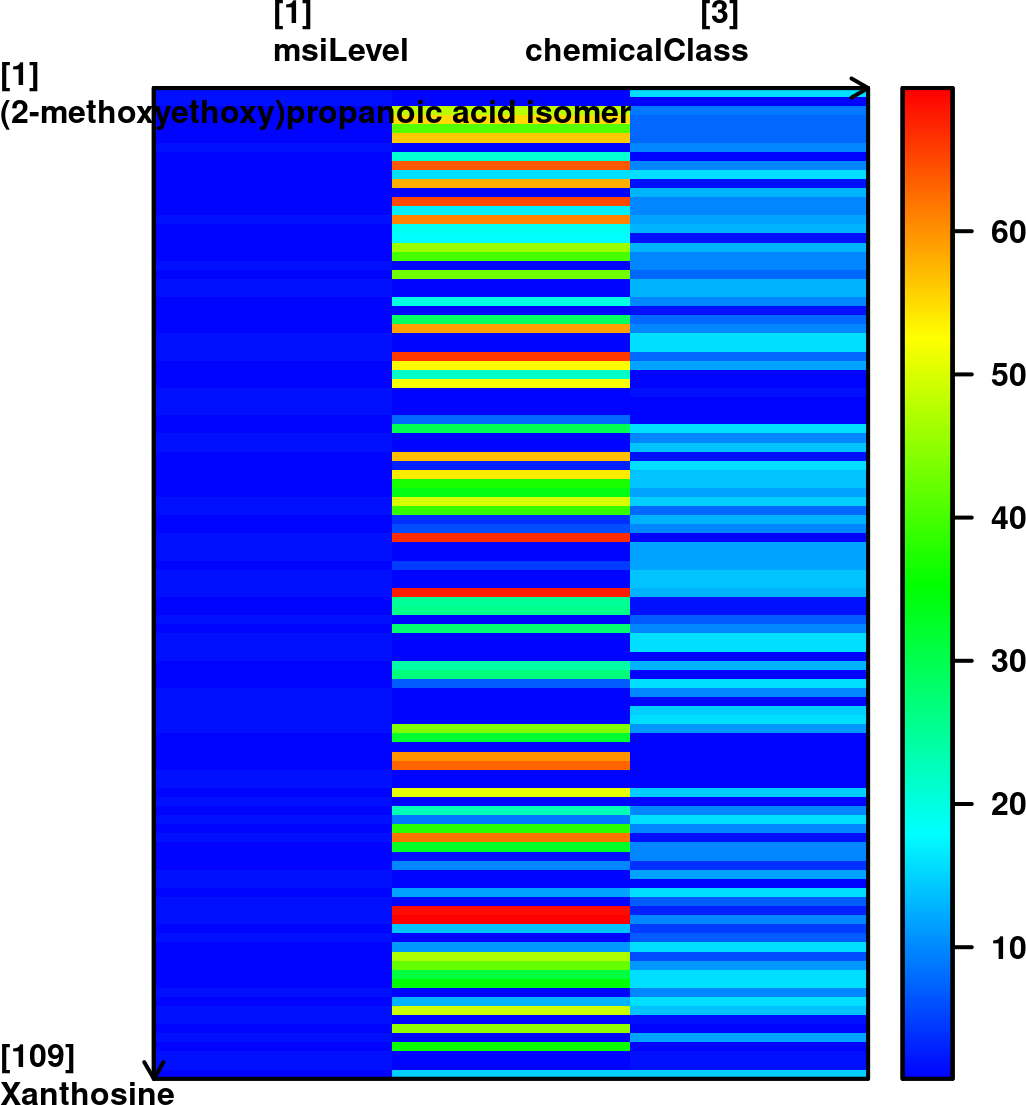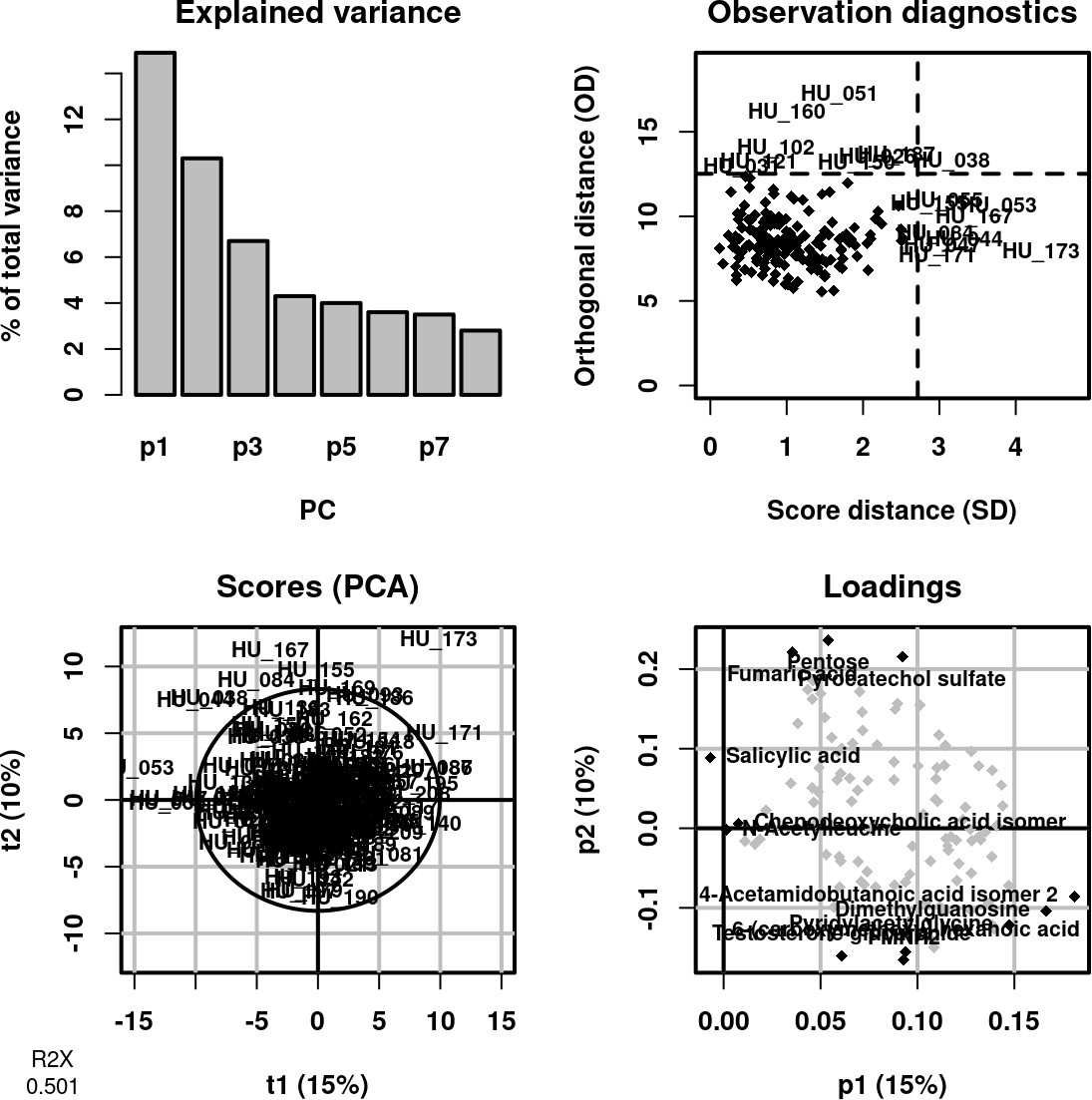# 1 The ropls package

The ropls R package implements the PCA, PLS(-DA) and OPLS(-DA) approaches with the original, NIPALS-based, versions of the algorithms (???). It includes the R2 and Q2 quality metrics (???), the permutation diagnostics (???), the computation of the VIP values (???), the score and orthogonal distances to detect outliers (???), as well as many graphics (scores, loadings, predictions, diagnostics, outliers, etc).

The functionalities from ropls can also be accessed via a graphical user interface in the Multivariate module from the Workflow4Metabolomics.org online resource for computational metabolomics, which provides a user-friendly, Galaxy-based environment for data pre-processing, statistical analysis, and annotation (???).

# 2 Context

## 2.1 Orthogonal Partial Least-Squares

Partial Least-Squares (PLS), which is a latent variable regression method based on covariance between the predictors and the response, has been shown to efficiently handle datasets with multi-collinear predictors, as in the case of spectrometry measurements (???). More recently, (???) introduced the Orthogonal Partial Least-Squares (OPLS) algorithm to model separately the variations of the predictors correlated and orthogonal to the response.

OPLS has a similar predictive capacity compared to PLS and improves the interpretation of the predictive components and of the systematic variation (???). In particular, OPLS modeling of single responses only requires one predictive component.

Diagnostics such as the Q2Y metrics and permutation testing are of high importance to avoid overfitting and assess the statistical significance of the model. The Variable Importance in Projection (VIP), which reflects both the loading weights for each component and the variability of the response explained by this component (???; ???), can be used for feature selection (???; ???).

## 2.2 OPLS software

OPLS is available in the SIMCA-P commercial software (Umetrics, Umea, Sweden; (???)). In addition, the kernel-based version of OPLS (???) is available in the open-source R statistical environment (???), and a single implementation of the linear algorithm in R has been described recently (???).

# 3 The sacurine metabolomics dataset

## 3.1 Study objective

The objective was to study the influence of age, body mass index (bmi), and gender on metabolite concentrations in urine, by analysing 183 samples from a cohort of adults with liquid chromatography coupled to high-resolution mass spectrometry (LC-HRMS; (???)).

## 3.2 Pre-processing and annotation

Urine samples were analyzed by using an LTQ Orbitrap in the negative ionization mode. A total of 109 metabolites were identified or annotated at the MSI level 1 or 2. After retention time alignment with XCMS, peaks were integrated with Quan Browser. Signal drift and batch effect were corrected, and each urine profile was normalized to the osmolality of the sample. Finally, the data were log10 transformed (???).

## 3.3 Covariates

The volunteers’ age, body mass index (bmi), and gender were recorded.

# 4 Hands-on

We first load the ropls package:

``library(ropls)``

We then load the sacurine dataset which contains:

1. The dataMatrix matrix of numeric type containing the intensity profiles (log10 transformed),

``````data(sacurine)
names(sacurine)``````
``##  "dataMatrix"       "sampleMetadata"   "variableMetadata"``

We attach sacurine to the search path and display a summary of the content of the dataMatrix, sampleMetadata and variableMetadata with the `view` method from the ropls package:

``attach(sacurine)``
``view(dataMatrix)``
``````##        dim  class    mode typeof   size NAs  min mean median max
##  183 x 109 matrix numeric double 0.2 Mb   0 -0.3  4.2    4.3   6
##        (2-methoxyethoxy)propanoic acid isomer (gamma)Glu-Leu/Ile ...
## HU_011                            3.019766011        3.888479324 ...
## HU_014                             3.81433889        4.277148905 ...
## ...                                       ...                ... ...
## HU_208                            3.748127215        4.523763202 ...
## HU_209                            4.208859398        4.675880567 ...
##        Valerylglycine isomer 2  Xanthosine
## HU_011             3.889078716 4.075879575
## HU_014             4.181765852 4.195761901
## ...                        ...         ...
## HU_208             4.634338821 4.487781609
## HU_209              4.47194762 4.222953354````````view(sampleMetadata)``
``````##      age     bmi gender
##  numeric numeric factor
##  nRow nCol size NAs
##   183    3 0 Mb   0
##         age   bmi gender
## HU_011   29 19.75      M
## HU_014   59 22.64      F
## ...     ...   ...    ...
## HU_208   27 18.61      F
## HU_209 17.5 21.48      F``````
``## 1 data.frame 'factor' column(s) converted to 'numeric' for plotting.````view(variableMetadata)``
``````##  msiLevel      hmdb chemicalClass
##   numeric character     character
##  nRow nCol size NAs
##   109    3 0 Mb   0
##                                        msiLevel      hmdb chemicalClass
## (2-methoxyethoxy)propanoic acid isomer        2                  Organi
## (gamma)Glu-Leu/Ile                            2                  AA-pep
## ...                                         ...       ...           ...
## Valerylglycine isomer 2                       2           AA-pep:AcyGly
## Xanthosine                                    1 HMDB00299        Nucleo``````
``## 2 data.frame 'character' column(s) converted to 'numeric' for plotting.``Note:

1. the `view` method applied to a numeric matrix also generates a graphical display

2. the `view` method can also be applied to an ExpressionSet object (see below)

## 4.2 Principal Component Analysis (PCA)

We perform a PCA on the dataMatrix matrix (samples as rows, variables as columns), with the `opls` method:

``sacurine.pca <- opls(dataMatrix)``

A summary of the model (8 components were selected) is printed:

``````## PCA
## 183 samples x 109 variables
## standard scaling of predictors
##       R2X(cum) pre ort
## Total    0.501   8   0``````

In addition the default summary figure is displayed:Figure 1: PCA summary plot. Top left `overview`: the scree plot (i.e., inertia barplot) suggests that 3 components may be sufficient to capture most of the inertia; Top right `outlier`: this graphics shows the distances within and orthogonal to the projection plane (???): the name of the samples with a high value for at least one of the distances are indicated; Bottom left `x-score`: the variance along each axis equals the variance captured by each component: it therefore depends on the total variance of the dataMatrix X and of the percentage of this variance captured by the component (indicated in the labels); it decreases when going from one component to a component with higher indice; Bottom right `x-loading`: the 3 variables with most extreme values (positive and negative) for each loading are black colored and labeled.

Note:

1. Since dataMatrix does not contain missing value, the singular value decomposition was used by default; NIPALS can be selected with the `algoC` argument specifying the algorithm (Character),

2. The `predI = NA` default number of predictive components (Integer) for PCA means that components (up to 10) will be computed until the cumulative variance exceeds 50%. If the 50% have not been reached at the 10th component, a warning message will be issued (you can still compute the following components by specifying the `predI` value).

Let us see if we notice any partition according to gender, by labeling/coloring the samples according to gender (`parAsColFcVn`) and drawing the Mahalanobis ellipses for the male and female subgroups (`parEllipseL`).

``````genderFc <- sampleMetadata[, "gender"]
plot(sacurine.pca,
typeVc = "x-score",
parAsColFcVn = genderFc)``````